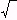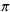Create a new printable
Answer key also includes questionsGeometry
Math Worksheets

Sample - Click above to make a new math worksheet (PDF).
 Name _____________________________Date ___________________
Perimeter and Area
Complete.
 1 What is the area of a square with perimeter 112 m?
 2 * This is a pre-made sheet.Use the link at the top of the page for a printable page.

Find the area.
 3 Find the area of the parallelogram whose vertices are(-6, -2), (-6, 0), (-2, 0), and (-2, -2)
 4 Find the area of the parallelogram whose vertices are(-5, -4), (-6, 0), (0, -4), and (-1, 0)

Complete.
 5 If the height of a rectangle is 2 1/4 cm and the area is 14 1/4 cm2, what is the length of the base of the rectangle?
 6 If the base of a rectangle is 5 2/5 cm and the area is 19 4/5 cm2, what is the height of the rectangle?

Complete.
 7 The area of a rectangle is 660 mm2. The height is five more than five times the base. What is the perimeter?
 8 The area of a rectangle is 68 mm2. The base is one more than four times the height. What is the height?

 Key #2
 aKey #2
Complete.
 9 What is the height of a triangle with base 24 cm and area 120 cm2?
 10 What is the area of a triangle with base 21 mm and height 11 mm?

Find the missing measurement of each trapezoid.
11.
 height = 5 m b1 = 21 m b2 = ________ area = 112.5 m2

12.
 height = ________ b1 = 14 cm b2 = 7 cm area = 115.5 cm2

13.
 height = 7.9 cm b1 = 12.2 cm b2 = 28.1 cm area = ________

Find the area.
 14 Find the area of the triangle whose vertices are(1, -6), (8, -6), and (1, -3)
 15 Find the area of the triangle whose vertices are(0, 9), (0, 5), and (4, 9)

Find the area.
 16 Find the area of the parallelogram whose vertices are(-2, -8), (-4, -3), (-1, -3), and (1, -8)
 17 Find the area of the square whose vertices are(6, -2), (2, -2), (6, 3), and (2, 3)

 Key #2
 aKey #2
Find the area.
 18 Find the area of the trapezoid whose vertices are(2, -2), (2, 2), (7, 2), and (9, -2)
 19 Find the area of the trapezoid whose vertices are(-8, 4), (3, 4), (0, 1), and (3, 1)

Complete.
 20 What is the length of the base of a triangle with height 3 1/3 cm and area 16 1/9 cm2?
 21 What is the area of a triangle with base 3 cm and height 9 1/2 cm?

Find the missing measurement of each trapezoid.
22.
 height = 6 1/3 cm b1 = 8 1/2 cm b2 = 2 cm area = ________

23.
 height = ________ b1 = 7 cm b2 = 7 3/5 cm area = 39 21/50 cm2

24.
 height = 9 cm b1 = ________ b2 = 6 3/4 cm area = 53 5/8 cm2

Find the missing length for each right triangle. Simplify your answer.
25.
a  =  18
b  =  ________
c  =
2202

26.
a  =  ________
b  =  14
c  =
713

27.
 a = 28 b = ________ c = 53

 Key #2
 aKey #2
Find the circumference of each circle. State your answer in terms ofand also round your answer to the nearest tenth.
28.
 diameter = 18.56 m

29.
 radius = 10 cm

30.
diameter =
 71
m

Find the area of each circle. State your answer in terms ofand also round your answer to the nearest tenth.
31.
 radius = 17 m

32.
 97
mm

33.
 diameter = 50.76 cm

Find the radius of the circle. (use= 3.14)
34.
 C  = 56.52 cm

35.
 C  = 44mm

36.
 A  = 0.56mm2

Find the area.
 37 Find the area of the triangle whose vertices are(3, -2), (7, -2), and (3, 4)
 38 Find the area of the rectangle whose vertices are(0, -6), (-9, -2), (-9, -6), and (0, -2)

 Key #2
 aKey #2
Complete.
 39 Stephanie wants to replace the baseboards around the recreation room in her house. If the room is seven and one-seventh feet by five and a half feet, and the material she is interested in using costs \$3.49 per foot, how much will it cost to buy the new baseboard material?
 40 A new crop circle has appeared in Cool Town. It is a rather simple one. It consists of two concentric circles. The largest (outer) circle has a radius of fifty meters and the inner circle has a radius of twenty-five meters. What is the difference between the perimeters of the circles?

Complete.
 41 How many tiles that are each one foot square are needed to cover a kitchen floor if the kitchen is eight feet long and nine feet wide?
 42 If a piece of paper is folded in half two times and the resulting folded paper is a square with an area of thirty-six square centimeters, what were the dimensions (length and width) of the piece of paper before it was folded?